Next: The NBODY1 Algorithm Up: N-body Algorithm Previous: N-body Algorithm

## Small-N Systems

In the study of the LG, the number of particles is well within the limit of the PP method, but as seen in the previous section, this method is rather slow. Apart from the softening parameter, are there any other ways to speed things up?

Aa-63 proposed a method known as the individual time-step scheme. The idea is that each particle is treated separately in the integration. The only coupling occurs at the time when the force on a particle is calculated. Also, the assignment of a time-step to each particle avoids the calculation of the force on every particle when only the recalculation of the force on a particular particle is required. This is especially important during close encounters when the force on a particle is changing very quickly and, hence, necessitates recalculation at much higher frequency than for the rest of the system. An improvement to this scheme has been made, called ``double individual'' time-steps. One then divide the force acting on a particle into two parts: one slowly varying part due to the ``distant'' particles, and a more rapid varying part due to particles in the immediate neighbourhood. This modification to the individual time-step scheme has not been adapted into Sverre Aarseth's N-body code NBODY1, which I will make use of, and will therefore not be discussed any further.

The basic idea of the method used in NBODY1, is that one calculates the force acting on a particle at a given time t by extrapolating from the forces, and its derivatives, calculated at earlier times. The particles will be assigned with two types of coordinates: a primary and a secondary,and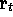. The secondary, evaluated at t, will be extrapolated from the primary, evaluated at. The same procedure is used for the velocities. The method of extrapolation used in this case is the Divided Difference Polynomial method.

Let us now take a closer look at this scheme Aa-85,AC. Mathematically, the N-body gravitational problem used in NBODY1 involves the solution of N second-order differential equations,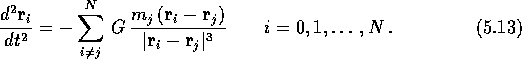The summation is over all particles of massand coordinates. This equation is only valid when the system in consideration is purely gravitational; i.e. that all other forces than gravitation can be ignored. To be able to compare NBODY1 to AVP, we need to modify this picture somewhat. In AVP, the cosmological constant play a major role for certain cosmological models, and has therefore a substantial effect on the orbits of galaxies in these models. This means that we have to introduce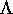to NBODY1. From the Poisson equation for a pressureless universe with a non-negligible cosmological constant, equation (3.17), we see that the modified version of equation (5.13) has to be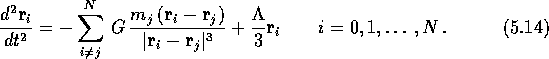The cosmological constant, or rather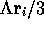, can be looked upon as a cosmic repulsive force, which counter the attractive gravitational force. Notice that equation (5.14) is the physical version of the Lagrangian in equation (3.20) solved in the AVP. The difference between AVP and N-body is therefore the way that these equations of motion are solved.

To avoid singularities as the mutual separation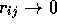, thereby making the numerical solution well behaved, and to reduce the computing time (see previous section), one can introduce a softening parameter c. Equation (5.14) then becomes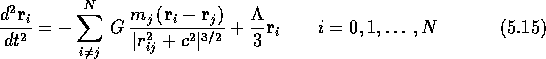where.

To evolve the positions and the velocities for a particle fromto t, a Taylor series up to the fourth order in the force derivative is used:where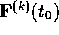is the kderivative of the force with respect to time, evaluated at time. The force is determined by extrapolating it from the values ofat four successive past epochs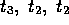, and, whereis the most recent. The fourth-order force polynomial is defined asThe three first divided differences are defined as (in compact notation)where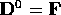and the square brackets refer to the appropriate time intervals. The term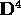is defined similarly byand.The force derivatives in terms of the divided differences are obtained by derivation of equation (5.18) at successive times and by setting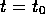. It should be noted that at, the fourth difference can not be determined due to it needing the knowledge of the force at the following time t. Thus, the contribution fromis included as an correcting factor at the end of each integration step. This so-called ``semi-iteration'' gives increased accuracy at little extra cost and no extra memory.Next: The NBODY1 Algorithm Up: N-body Algorithm Previous: N-body Algorithm

Trond Hjorteland
Mon Jul 5 02:59:28 MET DST 1999